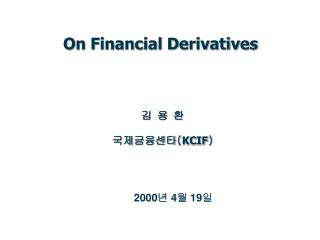# 2000 년 4 월 19 일 - PowerPoint PPT PresentationDownload Presentation2000 년 4 월 19 일

2000 년 4 월 19 일Download Presentation## 2000 년 4 월 19 일

- - - - - - - - - - - - - - - - - - - - - - - - - - - E N D - - - - - - - - - - - - - - - - - - - - - - - - - - -
##### Presentation Transcript

1. On Financial Derivatives 김 용 환 국제금융센타(KCIF) 2000년 4월 19일

2. <Scenario I > • Assume IBM price on 4/19/2000 to be x: • 4/19/2000 => Long IBM @100 • 4/19/2001 => Short IBM @x • 4/19/2001 => Profit and Loss = x – 100

3. <Scenario II > • [ Agreement ] • 4/19/2000 => Long IBM @100 • 4/19/2001 => Short IBM @x • 4/19/2001 => Finance Charge for 1-year = 10 • 4/19/2001 => Profit and Loss = x – (100+10)

4. <Scenario III > • [ Agreement ] • 4/19/2000 => Agreed to be Long IBM @y on 4/19/2001 • ( y is fixed on 4/19/2000 ) • 4/19/2001 => Profit and Loss = x – y • ( x is IBM price on 4/19/2001 ) • Note: if y = 110, • P&L of ScenarioII = P&L of ScenarioIII. • (profit and loss)

5. < Scenario IV > • Assume the finance charge to be 10% per annum: • 4/19/2000 => Borrow Cash y/1.1 • 4/19/2000 => Long IBM @100 • 4/19/2000 => Value = 100 – (y/1.1) • 4/19/2001 => Pay back Cash y • 4/19/2001 => Short IBM @x • 4/19/2001 => Profit and Loss = x – y – [100 – (y/1.1)] • Note: if y = 110, • P&L of Scenario II = P&L of Scenario IV.

6. <Expectation versus Arbitrage > • Kolmogrov’s Strong Law of Large Numbers • Let Xi’s be independent random numbers sampled from the same distribution with mean (expectation)  and let • S(n) = ( Xi)/n (arithmetic average). Then • lim S(n) =  • with probability one. • Example • Consider a game - tossing a coin and receiving \$1 for heads and nothing for tails. Fifty cents is a fair price of the game in the Strong Law sense.

7. Time Value of Money • Let t < T. Then the value at time t of a dollar promised at time T is given by • exp{–r(T– t)} • for some constantr > 0. The rate r is then the continuously compounded interest rate for this period. • Stock Model • Assume XN(, ). Then the natural logarithm of the stock price over some time period is given by X, i.e., • ln (ST) – ln (St) = X, or ST = Stexp(X)

8. Forward • Two parties enter into a contract whereby one agrees to give the other the stock at some agreed time(T) in the future in exchange for an amount(K) agreed now. • < Expectation Pricing for Forward > • By the strong law, • E[exp (–rT) (ST– K) ] = 0 • => K = E[ST] • => K = S0 exp(+0.52) • < Arbitrage Pricing for Forward > • Now: Borrow S0 and buy the stock with it and short forward at K • At T: Pay back S0 exp(rT) and settle forward at K • => K <= S0 exp(rT) and similarly we have K >= S0 exp(rT) • => K = S0 exp(rT)

9. Call Option • The buyer of a call option has the right to buy the underlying security on or before a specific date (T) at a specific price (K). Then the payoff = Max[ ST– K, 0 ]. Let C = C( t, S(t) ) denote the value of the option at time t. • < Arbitrage Pricing for European Call Option > • dS = S dt + S dW • dB = rB dt • dC = Ct dt + CS dS + 0.5 CSS dS dS • = [ Ct + SCS + 0.52S2CSS ] dt + SCS dW • Let  = C –S with  = CS , then • d= [ Ct + 0.52S2CSS ] dt • = r dt

10. We have the Black-Sholes partial differential equation • Ct + rSCS + 0.52S2CSS = rC • with the boundary condition, C( T, S(T) ) = payoff • < Martingale Pricing for European Call Option > • dS = S dt + S dW • dB = rB dt, letting Z = S/B, then • dZ = ( – r)Z dt + Z dW( by Ito’s lemma ) • letting  = ( – r)/, then • dW = dt + dW ( by Girsanov’s Theorem ) • dZ = Z dW <=> ZT = Z0 exp(–0.52t +  dWT) • C( 0, S(0) ) = E[ exp(– rT) payoff ]

11. < Fundamental Theorem of Derivative Asset Pricing > • There is a risk-neutral probability or an equivalent Martingalemeasure if and only if there is no arbitrage. • Also, there is a unique risk-neutral probability if and only if any European derivative asset can be hedged. In this case, the model is said to be complete.